# Calibration

The name of the current calibration file is displayed in the window header of the Calibration window. When displaying calibration curves saved in the chromatogram, the header contains the name of the chromatogram and the date the displayed calibration curves were saved. The window contains the main Compounds tab as well as tabs of every compound which is defined in the displayed calibration file. The Compounds tab contains the main calibration table and, eventually, the current calibration standard. The Individual Compound tabs contain a table for the displayed compound, several parameters and its calibration curve.

## Click on any area of the image below to get relevant informationCalibration - Compounds

The window contains the main Compounds tab as well as tabs of every compound which is defined in the displayed calibration file. The Compounds tab contains the main calibration table (Calibration Summary Table) and, eventually, the chromatogram of the current calibration standard. The Individual Compound tabs contain a table for the displayed compound, several parameters and its calibration curve.

Compounds tab

Calibration Summary Table

When using a multi-detector measurement, the main calibration table in the header contains the name of the current signal (detector) for which the data is being displayed.

Certain columns (e.g. Compound Name, Reten. Time, Amount) are common for all signals and are displayed in black, other columns which are dependent on the corresponding signal are displayed in the color of the active signal.

 Serial number of compound according to retention time. This column is common for all signals. Used The Used column has the following functionality: It allows to prohibit the use of a selected compound - meaning that unchecked compound will not be used for quantification. It serves as a check that two compounds do not have the same retention time on one signal - if this situation occurs, user is notified via a message box. Only compounds with checked Used checkbox will be (re)calibrated. This column is individual for each signal. Note: This column is particularly useful in multi-detector chromatograms where each compound is assessed on one signal and can be removed from the results table on the remaining signals using this selector. Compound Name Name of the compound. This column is common for all signals. When a new compound is manually created there is no pre-filled any default name. Reten. Time Retention time of the compound. This column is common for all signals. When a new compound is created there is pre-filled default value 0. Search Window Defines whether the Left Window and Right Window content will be inserted in Abs (absolute - set in minutes) or Rel (relative - set in percents of the Reten. Time) values. When a new compound is created there is pre-filled value currently set by item Search Window in Calibration Options - Defaults tab. Left (Right) Window Defines the width of the left (right) window for identification of compounds (the value is set in minutes). Maximum width is 50 minutes. This column is common for all signals. When a new compound is created there are pre-filled values currently set by items Left Window and Right Window in Calibration Options - Defaults tab. Peak Selection Defines the way the correct peak is matched with the Retention time when several peaks are present in the same identification window. The options available are Nearest (closest possible to the selected retention time - default for standard peaks), First, Last and Biggest (default for Reference peaks). Note: The Peak Selection choice is overridden whenever a peak is forced-linked to a compound in the calibration by the Set Peak Name function in the Chromatogram window. For more details see also chapter Peak. Peak Type Type of the calibrated compound. This column is common for all signals. The possible options are:Ordnr - Peak which is not a reference peak.Refer - Reference peak.Grp_ - Group consists of peaks from the chromatogram standard.Named Grp - Named group consists of peaks already present in the Calibration Summary Table.When a new compound is created, the default value is Ordnr. Such compound can be changed to Refer but not to Grp nor Named Grp.When a new group is created, the default value is Grp_ + a letter (A-Z) where the letter originates from the chromatogram of standard.When a new named group is created, the default value is Named Grp. Once created, Grp_ nor Named Grp cannot be changed to any other type - the cell is disabled. Is ISTD Defines whether the given compound is ISTD peak for the purposes of ISTD calculation and assigns the ISTD peak number to ISTD peaks. Up to 10 compounds in the calibration file may be indicated as internal standards from Clarity version 5.0 - before that only one ISTD peak was possible. When a new compound is created there is pre-filled default value None. Use ISTD Sets which ISTD peak will be used for the calculations of the given compound. When first ISTD peak is selected in the Is ISTD column of the calibration file, the Use ISTD column of all other compounds is automatically pre-filled with that peak name. ISTD peaks themselves have the Use ISTD column empty. Note: A group of peaks can be neither an internal standard nor reference peak. Peak Color Sets the coloration of the peak area to the desired color. Whenever the given peak will be identified in the chromatogram using this calibration, it will be colored accordingly. When a new compound is created there is pre-filled default color corresponding to RGB color code 255,255,255. Reten. Index Retention (Kovats) indexes. When a new compound is created there is pre-filled default value 0. Note: This column is hidden in the default layout. For more details see also chapter Setup Columns. LOD Limit of Detection (LOD) - the exceeding of the limits can be displayed in the result table. This column is individual for each signal. When a new compound is created there is pre-filled default value 0. LOQ Limit of Quantification (LOQ) - the exceeding of the limits can be displayed in the result table. This column is individual for each signal. When a new compound is created there is pre-filled default value 0. Response Base Response Base - Calculations are displayed for height - H (Height), area - A (Area) or area % - P (Area Percent) . This column is individual for each signal. When a new compound is created there is pre-filled value currently set by item Response Base in Calibration Options - Defaults tab. Resp. Factor Global response coefficient for free calibration. The value must be entered manually (not automatically calculated) and is used upon a calibrated calculation for compounds which have the flag filed Curve Fit Type set to Free Calibration. This column is individual for each signal. When a new compound is created there is pre-filled default value 0. Correction Factor Allows for the correction of amount due to the lower recovery etc. The Amount value is multiplied by the Correction Factor to calculate the final Amount. When a new compound is created there is pre-filled default value 1. Calculate By Clicking the field of this column on a given row opens the Calculate By dialog, which allows to set a compound whose Response will be used for Amount calculation for a given compound. By default this column is empty. Note: Named Group cannot be selected as Calculate By parameter. Curve Fit Type Determines the type of the curve used to fit the data - the available options are described in the section Curve Fit Type. When a new compound is created there is pre-filled value currently set by item Curve Fit Type in Calibration Options - Defaults tab. Origin Specifies the method for including origin zero point into the calibration curve. This value is individual for each signal and is described in the section Origin. When a new compound is created there is pre-filled value currently set by item Origin in Calibration Options - Defaults tab. Ending Point Specifies the maximum interpolation of Sigmoid and Half Sigmoid Curve Fit Type. The column is only editable when either of these Curve Fit Type options is selected and is described in the section Ending Point. When a new compound with these two Curve Fit Types is created default value is Last Point. Weighting Method Determines the value of the weight of individual points when calculating the interposing curve. The function and its possible options are described in the section Weighting Method. When a new compound is created there is pre-filled value currently set by item Weighting Method in Calibration Options - Defaults tab. Linearization X Sets the X-axis linearization for the calibration curve calculation. The function and its possible options are described in the section Linearization X. When a new compound is created there is pre-filled value currently set by item Linearization X in Calibration Options - Defaults tab. Linearization Y Sets the Y-axis linearization for the calibration curve calculation. The function and its possible options are described in the section Linearization Y. When a new compound is created there is pre-filled value currently set by item Linearization Y in Calibration Options - Defaults tab. Level X Number of the calibration level to which the following columns relate. This column is common for all signals. Note: Calibration table can contain up to 21 calibration levels including the blank. Response Area or height of peak as a response. Type is determined by the RB column. This column is individual for each signal. When a new compound is created there is pre-filled default value 0. Amount Amount of compound on the level displayed. Units are determined by the Units - Compound field from the Calibration Options dialog and are common for the entire calibration file. This column is common for all signals. Note: On any level other than 21 (blank), zero in the response or amount columns excludes the level from the calculation. Default Amount for blank level is Blank, default value for all other levels is 0. In case of combination Standard Addition Display Mode and Unknown level there is pre-filled default value Unknown. Standard Addition Display Mode is described in the STDADD section the chapter "Calibration Options" Resp. Fact. Response coefficient for each level. A more detailed description is provided below with the description of the compound table. This column is individual for each signal. When a new compound is created there is pre-filled default value 0. Rec No. Shows number of used recalibrations to number of total recalibrations on the level displayed. After the recalibration with Replace type, the number is set to 1/1. This column is individual for each signal. Clickinginvokes the Details of Calibration Point dialog with a detailed log of the selected level of the calibration point. When a new compound is created there is pre-filled default value 0/0. Note: A double-click on the row header with the number of compound (gray field to the left next to the name of the compound) displays the tab of the corresponding compound. Lin Response Displays calculated value of Response; the calculation is based on the option selected in Linearization Y. When a new compound is created there is pre-filled default value 0. Note: This column is hidden in the default layout. For more details see also chapter Setup Columns. Lin Amount Displays calculated value of Amount; the calculation is based on the option selected in Linearization X. When a new compound is created there is pre-filled default value 0. Note: This column is hidden in the default layout. For more details see also chapter Setup Columns.

Local menu of the Calibration Summary Table

In addition to the common commands for the majority of tables, the local menu contains the following items:

Delete Compound

Removes the actual substance from the calibration.

Delete All Compounds

Removes all compounds from the calibration (from all calibration levels).

Graph of the calibration standard

The graph refers to the calibration standard loaded by the File - Open Standard command. Its name is shown at the bottom right of the Status Bar and in the upper right corner of the graph. The graph facilitates the transfer of retention times and responses from the calibration standard to the calibration table, and is the same as the chromatogram graph in the Chromatogram window, including the characteristics set up by the Properties… command from the Display menu or the local menu. The only difference is the Peak Area Coloring area referring to the Calibration Table.

Note:

To see the colored peaks in the Calibration window, the calibration standard must have the current calibration linked to it (this can be done in the Chromatogram - Results tab).

Tabs of individual compounds: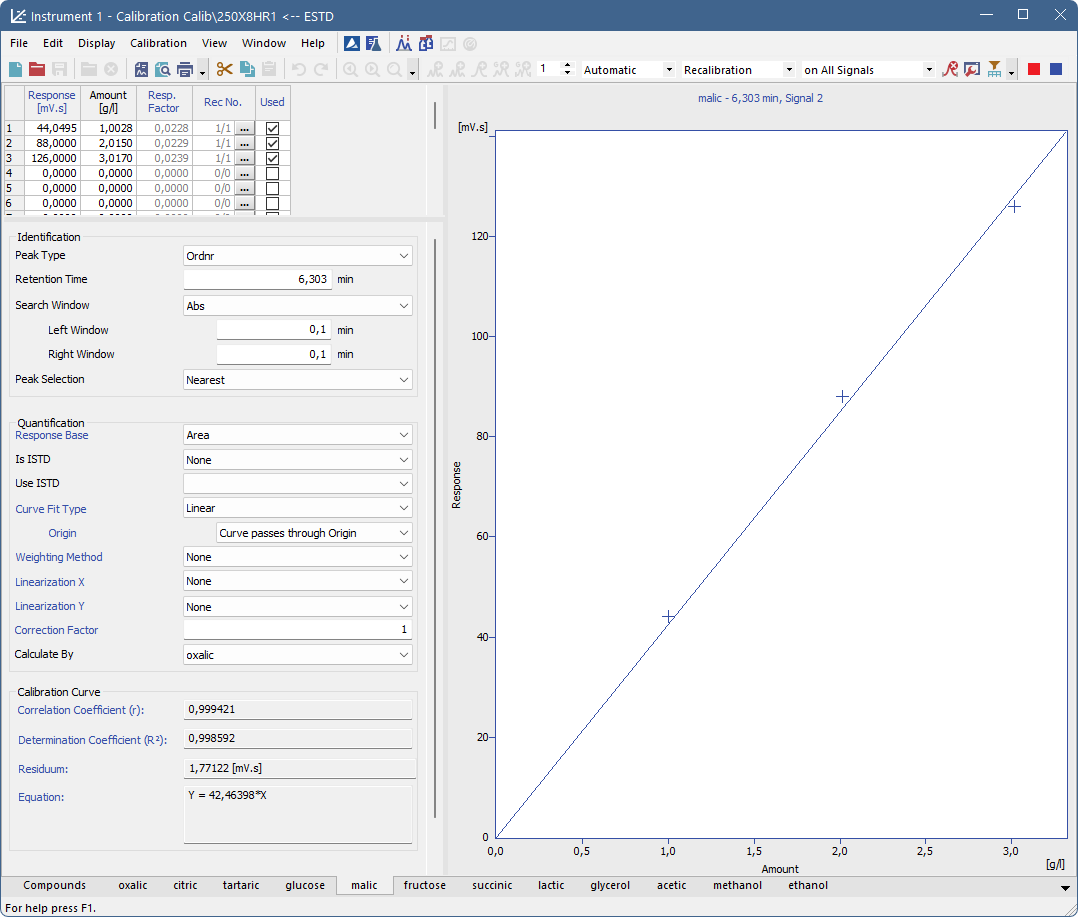Calibration - Individual Compound (oxalic)

Compound Table

The table can be found in the top left corner of the individual compound tab.

First column

Contains the level number of each displayed line.

Note:

Calibration table can contain up to 21 calibration levels, including blank.

Response

Represents the response at a given level. Whether it will be area or height is defined in the Response Base field.

Amount

The amount of the compound at a given level.

Response Factor

The response factor at a given level is equal to the reciprocal value of the slope of a straight line passing through the origin and the calibration point at the given level. It is calculated as the amount of the compound divided into the response at the relevant level. The value serves for information only.where:

Ai - is the amount of the compound at level i.

Ri - is the response (height or area) at level i.

It is possible to calculate response factor in inverse form ( RF = Response / Amount ) by checking Response Factor as Response / Amount checkbox in Calibration Options

Rec No.

Shows the number of recalibration points that have Used checkbox in the Details of Calibration Point checked to number of total recalibrations.

Used

Sets validity/invalidity of a calibration level for the purpose of the calibration curve. Cleared checkbox means that the calibration level will not be used in the calibration curve. It will be illustrated by a circle in the graph.

Note:

To omit calibration of a level from a Sequence, clear the Used checkbox on the corresponding calibration level.

Deviation [%]

Deviation of the Amount of the calibration point from the calculated Amount given by the calibration curve. The column is hidden by default.

Deviation[%] = ((Amount-AmountC)/AmountC) * 100

where:

Amount - The actual Amount on the concentration level.

AmountC- The calculated Amount corresponding to the concentration level (calculated from the calibration curve).

RSTD [%]

Displays the relative standard deviation of averaged points used during the recalibration of the respective concentration level. The column is hidden by default.

Lin Response

Displays calculated value of Response; the calculation is based on the option selected in Linearization Y. The column is hidden by default.

Lin Amount

Displays calculated value of Amount; the calculation is based on the option selected in Linearization X. The column is hidden by default.

Local menu of the Compound Table

In addition to common commands available for the majority of tables, the local menu contains the

 Clear All Levels Erases all entered values on all levels of the given substance. Clear Selected Level Erases all values on the given level. Clear Selected Value Erases the value in the current field of the table. Delete Compound Removes the current substance from the calibration. Show Details Invokes the Details of Calibration Point dialog with a detailed log of the selected level of the calibration point. Dialog window provides following details: used, peak retention time, area, height, date of a (re)calibration and the name of the calibration standard.

Resettable parameters

The section with resettable parameters is positioned in the bottom left part of each compound tab, below the Compound Table, and is divided into three logical groups: Identification, Quantification and Calibration Curve.

Identification

Resettable parameters which state how will be the compound identified.

Peak Type

This setting is individual for each compound, but common for all signals.

Ordnr - Designates a peak or group that is neither a reference peak nor an internal standard.

Refer - Designates a reference peak.

Retention Time

Retention time of the compound.

Search Window

Defines whether the Left Window and Right Window content will be inserted in Abs (absolute - set in minutes) or Rel (relative - set in percents of the Reten. Time) values.

Peak Selection

Defines the way the correct peak is matched with the Retention time when several peaks are present in the same identification window.

Quantification

Resettable parameters which state how will be the compound quantified.

Response Base

Sets whether the response will be used as the base for calculation of the calibration curve, calibration dependency equation and two response factors and specifies the base to be displayed in the Response column. This setting is individual for each signal. Please note that the height is calculated as the distance from the peak maximum vertically to the peak baseline, so for the small peaks on very steep baselines, the peak height may be imprecise.

Area - Peak area constitutes the base.

Height - Peak height is the base.

Area Percent - Calibration based of area percentage of peaks. Only peaks used in calibration are summed into the whole (100%), thus it is required to add them all into calibration, even when user don't need to calculate their results. Recommended type of calculation is ESTD.

Note:

Other fields from the tabs of individual compounds are dependent on the Response Base selected - switching the Response Base will thus switch the content of all editable fields to the set of saved parameters connected to the other Response Base option, the graph display will change accordingly and the content of the Response column in the Compound Table will change too.

Is ISTD

Defines whether the given compound is ISTD peak for the purposes of ISTD calculation.

Use ISTD

Sets which ISTD peak will be used for the calculations of the given compound. When first ISTD peak is selected in the Is ISTD column of the calibration file, the Use ISTD column of all other compounds is automatically pre-filled with that peak name. ISTD peaks themselves have the Use ISTD column empty.

Curve Fit Type

Determines the type of the curve used to fit the data:

Free Calibration - No calibration curve is construed and calibration equation is in the form of Y = Manual Response Factor * Correction Factor * X. Instead of a value read off the calibration curve the calculations use the corresponding global Response Factor from the global calibration table.

Point to Point - The calibration points are connected by a broken line. No common calibration equation is calculated, the equation of the relevant line segment is used instead in the calibration calculations.

Linear - A straight line is passed through the points.

Quadratic - The calibration curve will be interlined by a quadratic polynomial.

Cubic - The calibration curve will be interlined by a cubic polynomial.

Sigmoid - The calibration curve will be interlined by a sigmoid.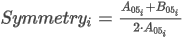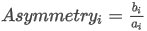c - Maximum Amount

d - Maximum Response

The c and d parameters can be increased up to 125% using the Ending Point field (both parameters are changed at once).

b = 1

a, k - are coefficients that are calculated using iteration from the interval <0,1 - 100> so that the curve will best fit the given calibration points.

ln - The calibration curve will be interlined by a logarithm function.

log10 - The calibration curve will be interlined by a decimal logarithm function.

exp - The calibration curve will be interlined by an exponent line.

pow10 - The calibration curve will be interlined by a decimal exponent line.

Hyperbola - The calibration curve will be interlined by a hyperbola.

Half Sigmoid - The calibration curve will be interlined by a half-sigmoid.

If the number of available points does not suffice for the required curve type, the curve will automatically be changed to the next lower type. The Curve Fit Type selection remains unchanged.

Note:

The Curve Fit Type selection is disabled in the Standard Addition display mode - only Linear curve fit is available in this mode.

Origin

Specifies the method for including origin zero point into the calibration curve. This value is individual for each signal.

Ignore Origin - Origin will not be included.

Compute with Origin - Considers the Origin to be one of the calibration points.

Curve passes through Origin - Always passes the Curve through the origin.

Note:

The Ignore Origin option is recommended for the Origin field when the blank response is included in the calibration curve calculation.

When interposing using the sigmoid, the Origin field will be replaced by the Ending Point field. In the Standard Addition display mode, the Origin Field is replaced with the Unknown field.

Ending Point

Enables the calculation of sigmoid interposing outside of the end points. The end calibration points are taken as limit values, increased by the relevant percentage according to the Ending Point field. Best used when the possibility to measure the calibration points within the sufficient range is limited.

Manual Response Factor

Serves as a manual amendment of a response for a whole compound and directly affects the constructed equation of the calibration curve. Manual Response Factor can only be changed when Curve Fit Type is set to Free Calibration.

Unknown

Specifies the method for including the unknown sample into the calibration curve calculation in the Standard Addition mode (for more details see also chapter Calibration Options). This value is individual for each signal.

Without Unknown Sample - Unknown sample will not be included in the calculation.

Compute with Unknown - Considers the Unknown sample to be one of the calibration points.

Curve passes through Unknown - Always passes the calibration curve through the origin.

Weighting Method

Determines the value of the weight of individual points when calculating the interposing curve. When weighting is not applied, points of higher concentrations (larger quantity and response) have a greater influence over the calibration curve than points with a lower concentration.

Weighting enables to apply the same relative influence to each point (1/x weighting method) or assign greater influence to points of lower levels (1/x^2 weighting method).

Weighting may be related to either the amount of the compound in the given point of the calibration curve (1/Amount or 1/Amount^2) or to the response of the compound in the given point of the calibration curve (1/Response or 1/Response^2).

Linearization X

Linearizes the values of the X-axis using the selected formula, and uses these linearized values to calculate the calibration curve equation. The X-axis and all calibration points are still displayed for the non-linearized values, so the interleaved curve will usually not match the calibration points.

Note:

To display the curve as a linear line, it is also necessary to check the Show Linearized Values checkbox in the Graph Properties dialog. For more details see also chapter Graph Properties.

Possible linearization formulas are None (no linearization), 1/Amount, ln(Amount), sqrt(Amount), 1/sqrt(Amount), Amount^2, 1/(Amount^2) and log(Amount).

Linearization Y

Linearizes the values of the Y-axis using the selected formula, and uses these linearized values to calculate the calibration curve equation. The Y-axis and all calibration points are still displayed for the non-linearized values, so the interleaved curve will usually not match the calibration points.

Note:

To display the curve as a linear line, it is also necessary to check the Show Linearized Values checkbox in the Graph Properties dialog. For more details see also chapter Graph Properties.

Possible linearization formulas are None (no linearization), 1/Response, ln(Response), sqrt(Response), 1/sqrt(Response), Response^2, 1/(Response^2) and log(Response).

Correction Factor

Allows for the correction of amount due to the lower recovery etc. For more details see described in the section Correction Factor.

Calculate By

Allows to set a compound whose Response will be used for Amount calculation for a given compound. For more details see described in the section Calculate By.

Calibration Curve - parameters

Parameters of calibration curve which are read only and cannot be modified directly here.

Correlation Factor

A number from the interval <0, 1> that characterizes the closeness-of-fit of the calibration curve. The closer is the number to 1, the better is fit of particular points to the calibration curve. The calculated value is individual for each signal.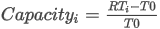where:

xi - the actual response at level i

yi - the calculated response at level i

x - the mean value of all actual responses (in case of Compute with Origin and Curve passes through Origin settings including zero point)

y - the mean value of all calculated responses (in case of Compute with Origin and Curve passes through Origin settings including zero point)

Residuum

The calculated value displays the calibration curve fit residuum calculated according to the following equation: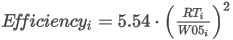where:

xi - the actual response at level i

yi - the calculated response at level i

n - number of calibration points (in case of Compute with Origin and Curve passes through Origin settings including zero point)

Equation

Contains the equation of the calibration curve, which determines the relationship between the response and the actual amount of the compound according to the curve type fit selected.

Curve Check

The calculated value displays the result of the linearity check for the calibration curve. The item is only displayed when the Curve Check option is set in the Calibration Options dialog. The result may be either OK (if all performed Curve Check tests are passed) or Error (if any of the performed tests is failed).

Calibration curve - graph

The calibration curve of any particular compound is located in the right-hand section of each compound tab.

Name of compound and retention time.

Shows the data which identify the displayed compound. These data come from the main calibration table and include Compound Name, Reten. Time and the name of the active signal.

Graph

Displays the calibration points of the current compound along with the calibration curve. The graph has identical properties to the other graphs, including cut-outs, auxiliary lines and optional setting using the Properties command from the local menu.

A calibrated point is indicated with a cross, a recalibrated point indicated with a star. In the STDADD calibration type, Blank sample is displayed as a vertical line along with multiplication cross sign to signal that the amount of the sample is not calculated from the intersection of the calibration curve with the X-axis on zero response, but from the intersection of the calibration curve with the blank horizontal line.

When using the ISTD method, a relative calibration curve will be displayed, depending on whether the same or a different amount of the internal standard has been used:

Same amount of internal standard

Relative calibration curve as dependence of response ratio of compound and internal standard on amount of injection of compound.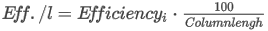Ri - response of i-th compound

RISTD - response of internal standard

Ai - amount of i-th compound

Different amount of internal standard

Relative calibration curve as dependence of response ratio of compound and internal standard on ratio of amount of compound and internal standard.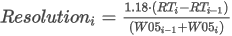Ri - response of i-th compound

RISTD - response of internal standard

Ai - amount of i-th compound

AISTD - amount of internal standard